Subscribe to our newsletter to receive the latest news and events from TWI:

# Radiography of Thin-Section Welds: Part 4 Further Modelling

## Radiography of Thin-Section Welds Part 4: Further Modelling

Charles R A Schneider
TWI, Cambridge CB1 6AL, UK

George A Georgiou
Jacobi Consulting Ltd
London N1 3NL, UK

Paper presented at BINDT Annual Conference 2004, Torquay, 14-16 Sept.

## Abstract

This paper describes the further refinement of a simple model of radiography, first published by Pollitt in 1962, which treats flaws as smooth, parallel-sided slots. It builds on the work on thin-section welds presented at NDT'02, and earlier work by TWI on thicker welds.

The latest experimental data were collected from 13 realistic planar flaws in welds of thicknesses 10-50mm. They were radiographed under various exposure conditions, giving 284 flaw/radiograph combinations (supplementing 333 flaw/radiograph combinations already available from earlier work on thicker welds). Each radiograph was evaluated 'blind' by two radiographers. The specimens were then sectioned to determine defect size, orientation and gape. A separate paper at this conference (Part 3) describes the practical aspects of the work.

The experimental data show variations in detectability that are strongly correlated with theoretical predictions. The broader range of the available experimental data has enabled us to improve the accuracy of our previously published statistical models for the reliability of radiography. Different models are proposed in the cases of:

• large planar flaws ( ≥15mm in through-wall extent) in thick-section welds,
• small planar flaws (<8mm through-wall) in thin-section welds, and
• small flaws of complex morphology in thin-section welds.

## 1. Introduction

Between 1995 and 1999, TWI performed several detailed studies on the radiography of large planar defects in thick-section welds.  This paper is based on an extension of the above work to investigate the detectability of planar defects in thinner section welds (thickness range 10-50mm). Extra sectioning information has been collected from the thin-wall specimens since the work was published at NDT'02, effectively superseding our earlier paper.  The work on thinner section welds, funded by the UK nuclear licensees, has specific applications in the nuclear power industry, but it is again believed to have generic value in improving the understanding of radiographic capability. The paper compares the experimental data with our earlier results from the thicker welds and shows how the new thin-wall data has enabled us to improve our previously published models.

## 2. Experimental programme

A separate paper at this conference provides a detailed account of the practical aspects of the work. 

As in our previous work,  the experimental results show variations in detectability with parameters such as penetrated thickness, gape and orientation, which are broadly in line with what is expected on simple theoretical arguments (e.g. decreasing detectability with increasing wall thickness). However, it is difficult to display the raw data in a form that enables a more quantitative comparison with theory. This is because of the large number of interrelated parameters that vary from defect to defect or exposure to exposure, making it difficult to isolate the effects of individual parameters.

## 3. Modelling

### 3.1 Pollitt model

As before,  we compared the experimental observations with the simple deterministic model of radiography described by Pollitt,  which treats planar defects as smooth, parallel-sided slots. The data continue to show variations in detectability similar to those reported previously. The results are again clearly correlated with the theoretical predictions, but less strongly for the thin-wall data than for the thick-wall data. Note that the theoretical treatment included all the refinements of the original model identified during our previous work. [2,4] These refinements were based mainly on ideas from Halmshaw, [6,7,8] and include (a) consideration of the geometrical enlargement of the radiographic image due to beam divergence and shot angle, and (b) a more rigorous treatment of radiographic unsharpness. Following these refinements, the deterministic model is either accurate or conservative in predicting all but three of the 593 thin-wall experimental results.

### 3.2 Theoretical index of detectability

TWI has developed a 'theoretical index' of detectability, which quantifies how easily a planar defect can be detected according to the Pollitt model. The theoretical index is positive if the Pollitt model predicts detection and negative if the Pollitt model predicts non-detection. For the data from the thick-section welds, we reported  an approximate relationship between the probability of defect detection p and the theoretical index I theory , given by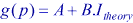(1)

where the so-called 'link function' g was given by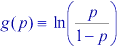(2)

The constant coefficients A and B in this model were estimated from the experimental data, by the maximum likelihood method, using a type of regression theory known as 'logistic' regression.  The particular form of the link function given by the right-hand side of equation (2) is called the 'logit' function.

To apply the maximum likelihood method, it is necessary to construct a function called the 'likelihood function'. This function expresses the probability of obtaining the observed set of data as a function of the unknown parameters A and B. The 'maximum likelihood estimators' of the parameters A and B are those values that maximise the likelihood function. In this respect, the resulting estimates of A and B are those that agree most closely with the observed data.  In general, it is easier to work with the logarithm of the likelihood function, which is referred to as the 'log-likelihood' function.

### 3.3 Tests for homogeneity between data sets

3.3.1 Do the thin-wall data follow the same trend as the thick-wall data?

One way of testing whether there is a statistical difference between the thin-wall and thick-wall data is to introduce a design variable W for the wall thickness, which is equal to one for the thin-wall data and zero for the thick-wall data, and then fit the following bivariate model: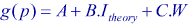(3)
The fitted model gave a value of C that is significant at the 15% level advocated in the literature.  This test shows that, for a given index of detectability, there is a significant difference between the probability of detection (POD) of defects in the thin-section welds and that in the thick-section welds. The above design variable is therefore included in the model to reflect this difference in POD.

It could be that these differences arise because:

• the faces of the flaws in the thick-wall data set tend to be rougher than those in the thin-wall data set (this is a speculative hypothesis, given that the roughness of the flaws in the thin welds have not been measured to date);
• the flaws in the thin-wall data have much smaller through-wall extents (<8mm through-wall) than the flaws in the thick-wall data set (which were all ≥15mm in through-wall extent).

Multivariate analysis of the thick-section data  previously showed that both of the above factors have an influence on radiographic detectability over and above that predicted by the detectability index.

3.3.2 Is the performance of Pollitt theory influenced by flaw 'complexity'?

A distinction was also made between flaws that were judged to have a 'complex' shape ( e.g. Figure 1), as opposed to flaws that were more akin to smooth, parallel-sided slots ( e.g. Figure 2), for which Pollitt theory is intuitively expected to perform better. We tested whether the difference between these 'complex' flaws and the 'simple' flaws was statistically significant, in a similar way to that described above, by introducing a further design variable Z. The coefficient of this design variable was also found to be significant at the 15% significance level. This suggests that Pollitt theory is, in some sense, influenced by how complex the morphology of the flaw is. As above, the corresponding design variable is accordingly included in the model.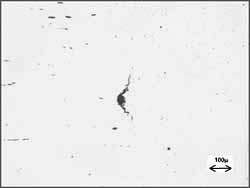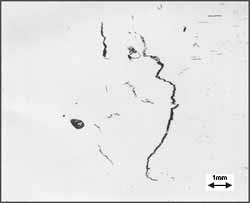Fig. 1. Examples of photographs of two 'complex' hydrogen cracks revealed by sectioning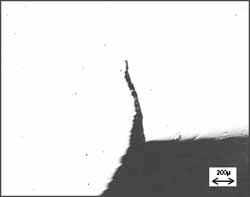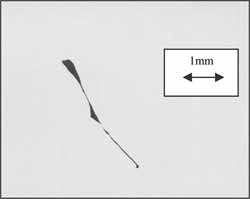Fig. 2. Examples of photographs of two 'simple' flaws revealed by sectioning:
a lack of root fusion flaw (Top), and a lack of side-wall fusion flaw (Below)

Note that all the flaws studied in the thick-section welds were judged to have a simple morphology. Thus, the two design variables W and Z introduced above divide the data into just three subsets, corresponding to:

• large planar flaws ( ≥15mm in through-wall extent) in thick-section welds,
• small planar flaws (<8mm through-wall) in thin-section welds, and
• small flaws of complex morphology in thin-section welds.

3.3.3 Tests for interactions

The two design variables W and Z effectively allow a constant shift in the value of the link function in equation (1) between the three different data sets. But the resulting model still assumes that the slope coefficient B is the same for each data set. The standard method  of testing the validity of this assumption is to include second order terms, called 'interactions', in the model and test whether the coefficients of these terms have statistically significant coefficients. In this case, the appropriate interaction terms are formed by creating the product of each of the above design variables with the index of detectability.

The interaction of the index with flaw shape was not found to be statistically significant (at the 15% level). However, the interaction of the index with the design variable W of Section 3.3.1 was significant (at the 15% level). This indicates that the slope coefficient B is significantly larger for the thick-wall data than for the thin-wall data, which means that the 'grey area' between detection and non-detection straddles a smaller range of values of the index for the thick-wall data. This may reflect the fact that more detailed sectioning data was available from the large flaws in the thick-section welds.

### 3.4 Choice of link function

The logit function of equation (2) is the most commonly used link function.  The Minitab statistical package allows two alternative link functions and recommends using whichever link function yields a good fit to the data. 

The first of these alternative link functions is called the 'gompit' (or complementary log-log) function, and is given by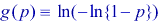(4)

The second of these alternative link functions is called the 'normit' (or probit) function, and is given by the inverse of the cumulative standard normal distribution function, i.e.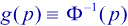(5)

The three link functions (2), (4) and (5) yield three different models. Standard goodness-of-fit tests  indicate that all three models provide an adequate fit to the experimental data (at the 5% significance level). However, the log-likelihood function is equal to -420.9 for the normit model, as opposed to -422.0 and 422.1 for the logit model and the gompit model respectively. These differences are relatively small and do not provide strong support for one link function over another. But, in the absence of any other evidence, they suggest a slight preference for the normit model. Thus the POD model finally selected takes the form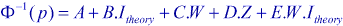(6)

where the coefficients A, B, C, D and E are estimated from the experimental data by the maximum likelihood method.

### 3.5 Tests for non-linearity

The goodness-of-fit tests above suggest that the linear model given by equation (1) is adequate. This finding can be further supported by means of a statistical test of non-linearity. This is done by adding a term of the form xln x to the model.  If the coefficient of this new variable is significant, this constitutes evidence of non-linearity in the logit with respect to x. By setting x = I + 7 (to avoid taking logs of a negative number), this test confirms that a linear model is adequate (at the 5% level).

Finally, we confirm the linearity of the normit model by grouping each of the three data sets identified in Section 3.3 according to the theoretical index I theory and plotting an estimate of the normit function given by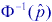, where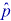is the proportion of detections within each group. The resulting plot is shown in Figure 3.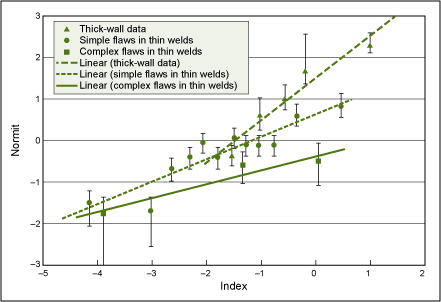Fig.3. Plots of estimated normit function against index of detectability

The error bars are based on estimates of the two-sided 80% confidence intervals for each data set. There are a total of 20 data points on this plot, so it is not surprising that four (20%) of the error bars fail to intersect the appropriate regression line. Overall, the plot confirms a broadly linear trend in the normit function for each data set. As expected, the two trend lines for the thin-wall data are approximately parallel, while the trend line for the thick-wall data clearly has a larger gradient.

Note that the regression lines plotted in Figure 3 are simply calculated by the method of least squares, and do not take into account the variation in the size of the error bars. They are included merely to assist our eyeball assessment of linearity.

## 4. Conclusions

The models described in this paper can be used to estimate how easily a large planar defect can be detected by radiography, from knowledge of its size, orientation and other relevant parameters. In principle, the probability of detection and an associated confidence interval can be estimated. The models apply to defects of a similar type to those we have examined experimentally; caution needs to be exercised in extrapolating the results to other defects.

### Acknowledgements

We gratefully acknowledge the support of Dr R K Chapman and Mr G S Woodcock of British Energy plc. The paper is published by permission of British Energy and BNFL who jointly funded the work.

### References

1. R K Chapman, G S Woodcock, I J Munns, C R A Schneider, G A Georgiou and A B Wooldridge, 'Recent experimental studies on radiographic capability on thick-section welds', NDT'99 proceedings.
2. C R A Schneider and G A Georgiou, 'The model approach to radiography', Bulletin November/December 2003. Also published (under different title) in Insight, February 2003, Vol.45 No 2, pp119-121.
3. G A Georgiou and C R A Schneider, 'Radiography of thin section welds, part 3: additional defect measurements'. Also to be presented at NDT 2004.
4. C R A Schneider and I J Munns, 'Improved models for the reliability of radiography of thick-section welds', NDT'99 proceedings.
5. C G Pollitt, 'Radiographic sensitivity', Brit. J. NDT, Vol 4, No 3, pp 71-80, September 1962.
6. R Halmshaw, 'Industrial radiology - theory and practice', Applied Science, London and New Jersey, 1982.
7. R Halmshaw, 'Industrial radiology', 1966 (earlier edition of (6) ).
8. R Halmshaw, 'The factors involved in an assessment of radiographic definition', J. Photographic Science, Vol 3, pp 161-168, 1955.
9. D W Hosmer and S Lemeshow, 'Applied logistic regression', John Wiley & Sons, New York, 1989.
10. Minitab reference manual - Release 12 for Windows. Minitab Inc. (USA), February 1998.